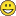# New to QlikView

Discussion board where members can get started with QlikView.

Highlighted
Contributor

## OR in set analysis

How to put OR between 2 dates in the below set analysis ?

(Count({<

[Activity Status] ={'Active'},

[ Plan] = {"\$(='>' & Date(Today()))"} or [Revised] = {"\$(='>' & Date(Today()))"}

>} [ID]) )

If I do as below few ID's will get counted twice...

(Count({<

[Activity Status] ={'Active'},

[ Plan] = {"\$(='>' & Date(Today()))"}

>} [ID]) )

+

(Count({<

[Activity Status] ={'Active'},

[Revised] = {"\$(='>' & Date(Today()))"}

>} [ID]) )

Thanks Much

2 RepliesMVP

## Re: OR in set analysis

May be like this:

Count({<[Activity Status] ={'Active'}, [ Plan] = {"\$(='>' & Date(Today()))"}>+<[Activity Status] ={'Active'}, [Revised] = {"\$(='>' & Date(Today()))"}>} [ID])

Try this (Sunny has helped me on a similar problem last week)